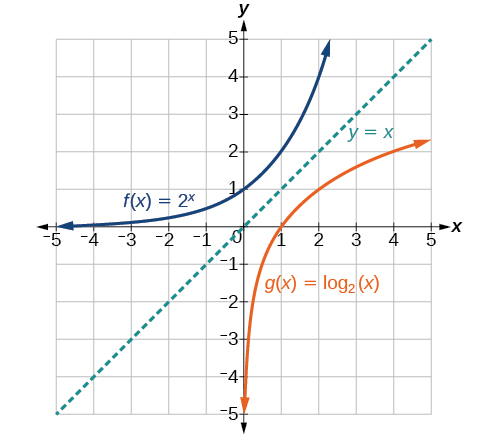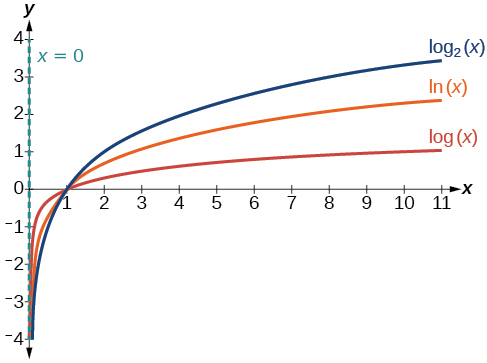# 6.4 Graphs of logarithmic functions  (Page 2/8)

 Page 2 / 8

Given a logarithmic function, identify the domain.

1. Set up an inequality showing the argument greater than zero.
2. Solve for $\text{\hspace{0.17em}}x.$
3. Write the domain in interval notation.

## Identifying the domain of a logarithmic shift

What is the domain of $\text{\hspace{0.17em}}f\left(x\right)={\mathrm{log}}_{2}\left(x+3\right)?$

The logarithmic function is defined only when the input is positive, so this function is defined when $\text{\hspace{0.17em}}x+3>0.\text{\hspace{0.17em}}$ Solving this inequality,

The domain of $\text{\hspace{0.17em}}f\left(x\right)={\mathrm{log}}_{2}\left(x+3\right)\text{\hspace{0.17em}}$ is $\text{\hspace{0.17em}}\left(-3,\infty \right).$

What is the domain of $\text{\hspace{0.17em}}f\left(x\right)={\mathrm{log}}_{5}\left(x-2\right)+1?$

$\left(2,\infty \right)$

## Identifying the domain of a logarithmic shift and reflection

What is the domain of $\text{\hspace{0.17em}}f\left(x\right)=\mathrm{log}\left(5-2x\right)?$

The logarithmic function is defined only when the input is positive, so this function is defined when $\text{\hspace{0.17em}}5–2x>0.\text{\hspace{0.17em}}$ Solving this inequality,

The domain of $\text{\hspace{0.17em}}f\left(x\right)=\mathrm{log}\left(5-2x\right)\text{\hspace{0.17em}}$ is $\text{\hspace{0.17em}}\left(–\infty ,\frac{5}{2}\right).$

What is the domain of $\text{\hspace{0.17em}}f\left(x\right)=\mathrm{log}\left(x-5\right)+2?$

$\left(5,\infty \right)$

## Graphing logarithmic functions

Now that we have a feel for the set of values for which a logarithmic function is defined, we move on to graphing logarithmic functions. The family of logarithmic functions includes the parent function $\text{\hspace{0.17em}}y={\mathrm{log}}_{b}\left(x\right)\text{\hspace{0.17em}}$ along with all its transformations: shifts, stretches, compressions, and reflections.

We begin with the parent function $\text{\hspace{0.17em}}y={\mathrm{log}}_{b}\left(x\right).\text{\hspace{0.17em}}$ Because every logarithmic function of this form is the inverse of an exponential function with the form $\text{\hspace{0.17em}}y={b}^{x},$ their graphs will be reflections of each other across the line $\text{\hspace{0.17em}}y=x.\text{\hspace{0.17em}}$ To illustrate this, we can observe the relationship between the input and output values of $\text{\hspace{0.17em}}y={2}^{x}\text{\hspace{0.17em}}$ and its equivalent $\text{\hspace{0.17em}}x={\mathrm{log}}_{2}\left(y\right)\text{\hspace{0.17em}}$ in [link] .

 $x$ $-3$ $-2$ $-1$ $0$ $1$ $2$ $3$ ${2}^{x}=y$ $\frac{1}{8}$ $\frac{1}{4}$ $\frac{1}{2}$ $1$ $2$ $4$ $8$ ${\mathrm{log}}_{2}\left(y\right)=x$ $-3$ $-2$ $-1$ $0$ $1$ $2$ $3$

Using the inputs and outputs from [link] , we can build another table to observe the relationship between points on the graphs of the inverse functions $\text{\hspace{0.17em}}f\left(x\right)={2}^{x}\text{\hspace{0.17em}}$ and $\text{\hspace{0.17em}}g\left(x\right)={\mathrm{log}}_{2}\left(x\right).\text{\hspace{0.17em}}$ See [link] .

 $f\left(x\right)={2}^{x}$ $\left(-3,\frac{1}{8}\right)$ $\left(-2,\frac{1}{4}\right)$ $\left(-1,\frac{1}{2}\right)$ $\left(0,1\right)$ $\left(1,2\right)$ $\left(2,4\right)$ $\left(3,8\right)$ $g\left(x\right)={\mathrm{log}}_{2}\left(x\right)$ $\left(\frac{1}{8},-3\right)$ $\left(\frac{1}{4},-2\right)$ $\left(\frac{1}{2},-1\right)$ $\left(1,0\right)$ $\left(2,1\right)$ $\left(4,2\right)$ $\left(8,3\right)$

As we’d expect, the x - and y -coordinates are reversed for the inverse functions. [link] shows the graph of $\text{\hspace{0.17em}}f\text{\hspace{0.17em}}$ and $\text{\hspace{0.17em}}g.$Notice that the graphs of   f ( x ) = 2 x   and   g ( x ) = log 2 ( x )   are reflections about the line   y = x .

Observe the following from the graph:

• $f\left(x\right)={2}^{x}\text{\hspace{0.17em}}$ has a y -intercept at $\text{\hspace{0.17em}}\left(0,1\right)\text{\hspace{0.17em}}$ and $\text{\hspace{0.17em}}g\left(x\right)={\mathrm{log}}_{2}\left(x\right)\text{\hspace{0.17em}}$ has an x - intercept at $\text{\hspace{0.17em}}\left(1,0\right).$
• The domain of $\text{\hspace{0.17em}}f\left(x\right)={2}^{x},$ $\left(-\infty ,\infty \right),$ is the same as the range of $\text{\hspace{0.17em}}g\left(x\right)={\mathrm{log}}_{2}\left(x\right).$
• The range of $\text{\hspace{0.17em}}f\left(x\right)={2}^{x},$ $\left(0,\infty \right),$ is the same as the domain of $\text{\hspace{0.17em}}g\left(x\right)={\mathrm{log}}_{2}\left(x\right).$

## Characteristics of the graph of the parent function, f ( x ) = log b ( x )

For any real number $\text{\hspace{0.17em}}x\text{\hspace{0.17em}}$ and constant $\text{\hspace{0.17em}}b>0,$ $b\ne 1,$ we can see the following characteristics in the graph of $\text{\hspace{0.17em}}f\left(x\right)={\mathrm{log}}_{b}\left(x\right):$

• one-to-one function
• vertical asymptote: $\text{\hspace{0.17em}}x=0$
• domain: $\text{\hspace{0.17em}}\left(0,\infty \right)$
• range: $\text{\hspace{0.17em}}\left(-\infty ,\infty \right)$
• x- intercept: $\text{\hspace{0.17em}}\left(1,0\right)\text{\hspace{0.17em}}$ and key point $\left(b,1\right)$
• y -intercept: none
• increasing if $\text{\hspace{0.17em}}b>1$
• decreasing if $\text{\hspace{0.17em}}0

[link] shows how changing the base $\text{\hspace{0.17em}}b\text{\hspace{0.17em}}$ in $\text{\hspace{0.17em}}f\left(x\right)={\mathrm{log}}_{b}\left(x\right)\text{\hspace{0.17em}}$ can affect the graphs. Observe that the graphs compress vertically as the value of the base increases. ( Note: recall that the function $\text{\hspace{0.17em}}\mathrm{ln}\left(x\right)\text{\hspace{0.17em}}$ has base $\text{\hspace{0.17em}}e\approx \text{2}.\text{718.)}$The graphs of three logarithmic functions with different bases, all greater than 1.

bsc F. y algebra and trigonometry pepper 2
given that x= 3/5 find sin 3x
4
DB
remove any signs and collect terms of -2(8a-3b-c)
-16a+6b+2c
Will
Joeval
(x2-2x+8)-4(x2-3x+5)
sorry
Miranda
x²-2x+9-4x²+12x-20 -3x²+10x+11
Miranda
x²-2x+9-4x²+12x-20 -3x²+10x+11
Miranda
(X2-2X+8)-4(X2-3X+5)=0 ?
master
The anwser is imaginary number if you want to know The anwser of the expression you must arrange The expression and use quadratic formula To find the answer
master
The anwser is imaginary number if you want to know The anwser of the expression you must arrange The expression and use quadratic formula To find the answer
master
Y
master
master
Soo sorry (5±Root11* i)/3
master
Mukhtar
explain and give four example of hyperbolic function
What is the correct rational algebraic expression of the given "a fraction whose denominator is 10 more than the numerator y?
y/y+10
Mr
Find nth derivative of eax sin (bx + c).
Find area common to the parabola y2 = 4ax and x2 = 4ay.
Anurag
A rectangular garden is 25ft wide. if its area is 1125ft, what is the length of the garden
to find the length I divide the area by the wide wich means 1125ft/25ft=45
Miranda
thanks
Jhovie
What do you call a relation where each element in the domain is related to only one value in the range by some rules?
A banana.
Yaona
given 4cot thither +3=0and 0°<thither <180° use a sketch to determine the value of the following a)cos thither
what are you up to?
nothing up todat yet
Miranda
hi
jai
hello
jai
Miranda Drice
jai
aap konsi country se ho
jai
which language is that
Miranda
I am living in india
jai
good
Miranda
what is the formula for calculating algebraic
I think the formula for calculating algebraic is the statement of the equality of two expression stimulate by a set of addition, multiplication, soustraction, division, raising to a power and extraction of Root. U believe by having those in the equation you will be in measure to calculate it
Miranda
state and prove Cayley hamilton therom
hello
Propessor
hi
Miranda
the Cayley hamilton Theorem state if A is a square matrix and if f(x) is its characterics polynomial then f(x)=0 in another ways evey square matrix is a root of its chatacteristics polynomial.
Miranda
hi
jai
hi Miranda
jai
thanks
Propessor
welcome
jai
What is algebra
algebra is a branch of the mathematics to calculate expressions follow.
Miranda
Miranda Drice would you mind teaching me mathematics? I think you are really good at math. I'm not good at it. In fact I hate it. 😅😅😅
Jeffrey
lolll who told you I'm good at it
Miranda
something seems to wispher me to my ear that u are good at it. lol
Jeffrey
lolllll if you say so
Miranda
but seriously, Im really bad at math. And I hate it. But you see, I downloaded this app two months ago hoping to master it.
Jeffrey
which grade are you in though
Miranda
oh woww I understand
Miranda
Jeffrey
Jeffrey
Miranda
how come you finished in college and you don't like math though
Miranda
gotta practice, holmie
Steve
if you never use it you won't be able to appreciate it
Steve
I don't know why. But Im trying to like it.
Jeffrey
yes steve. you're right
Jeffrey
so you better
Miranda
what is the solution of the given equation?
which equation
Miranda
I dont know. lol
Jeffrey
Miranda
JeffreyByByBy IES PortalBy OpenStaxBy OpenStaxBy Jazzycazz JacksonBy OpenStaxBy Jazzycazz JacksonBy Mary CohenBy Katy KeilersBy OpenStaxBy Brooke Delaney